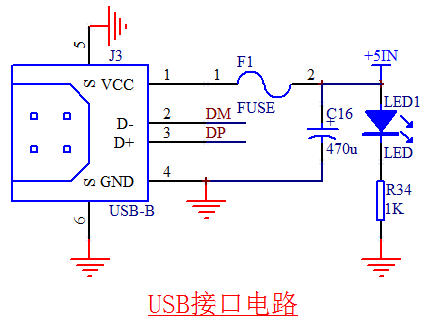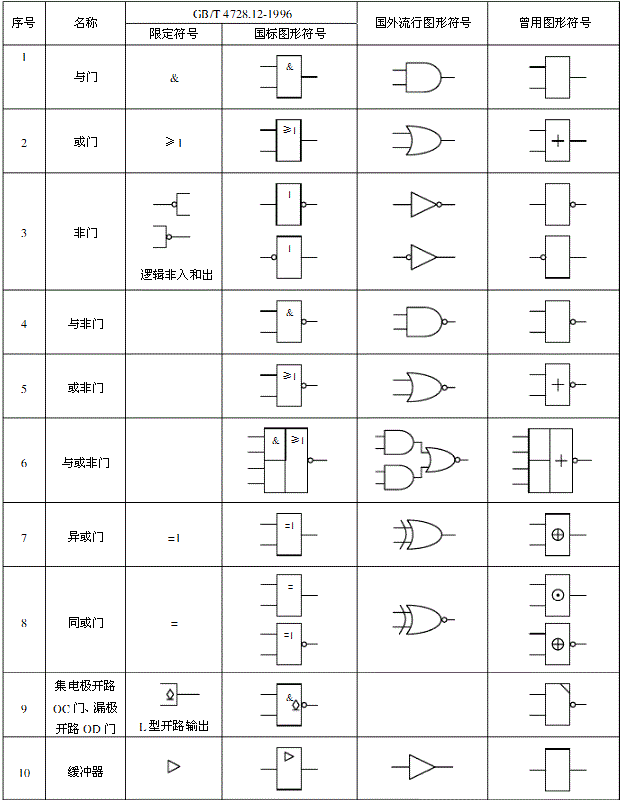# 单片机啤酒瓶计数实验源程序

2020-07-29来源: 51hei关键字：单片机  啤酒瓶计数实验  LED显示

QQ

#include

char led[]={0x3f,0x06,0x5b,0x4f,0x66,

0x6d,0x7d,0x07,0x7f,0x6f};  //led显示字模

sbit P37=P3^7;

sbit P34=P3^4;

void delay(unsigned int time)  //约4ms的延时函数

{

unsigned int j;

for (;time>0;time--);

for (j=0;j<500;j++);

}

void t0() interrupt 1

{

TR0=0;           //停止计数

P37=0;           //启动电机运转

delay(1000); //延时，等待下一空箱运转到位待装啤酒

P37=1;           //停止电机运转

TR0=1;           //启动计数

}

/**********主函数**************/

void main()

{

char i;

TMOD=0x06;                //T0计数方式

TL0=0xf7;                //计数初值247

TH0=0xf7;

TR0=1;                        //启动T0计数

ET0=1;                   //开中断

EA=1;

P37=1;                        //停止电机运转

while(1)

{

i=256-TL0;          //获取计数差值

P0=led[9-i];          //显示已装了多少啤酒

delay(1000);

if(i==1)                 //是否已经装了（9-1）=8瓶

{

while(P34==1);  //等待装最后一瓶

P0=0x71;                        //显示已经装满符号

delay(100);

}

}

}北京航空航天大学教授，20余年来致力于单片机与嵌入式系统推广工作。北京市海淀区知春路23号集成电路设计园量子银座1305 电话:(010)82350740 邮编：100191